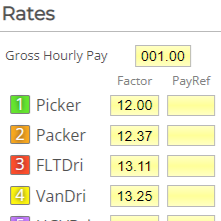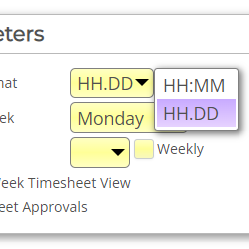# How do I assign different pay values to Rates?This is done by making adjustments to an employee’s Rate Factors and Gross Hourly Pay in their Employee Form. However, before we explain how to do this, it helps to know how Focus calculates pay. Having an understanding of how Rate Factors are traditionally used will also make the process of changing them much easier.

This guide will cover:

• How does Focus calculate pay?
• Rate Factors (standard use): Gross Hourly Pay as a monetary value
• Rate Factors (alternative use): Rate Factors as monetary values

## How does Focus calculate pay?

When Focus calculates how much an employee is paid, it does so using two equations.
The first calculates an employee’s Weekly Gross Total:

Hours worked x Rate Factor = Weekly Gross Total*

Behind the scenes, the second then calculates the employees pay:

Weekly Gross Total x Gross Hourly Pay = Gross Weekly Pay**

* It may help to simply view the Weekly Gross Total as a number. Depending on how you use Rate Factors, it could be seen as either an hours total or a monetary total.

** Gross Weekly Pay does not show anywhere in Focus. It is purely used here to help demonstrate how Focus calculates pay.

## Rate Factors (standard use):Gross Hourly Pay as a monetary value

### When is this method used?

Commonly used when an employee earns a set basic rate of pay for working their regular hours and earns differing percentages of their basic pay for working overtime / nights / weekends etc. These percentages are applied using Rate Factors.

A Rate Factor set to 01.00 means that an employee will receive 100% of their Gross Hourly Pay for any time worked at that Rate (basic rate of pay).

A Rate Factor set to 01.50 means that an employee will receive 150% of their Gross Hourly Pay for any time worked at that Rate (traditionally known as time and a half).

A Rate Factor set to 02.00 means that an employee will receive 200% of their Gross Hourly Pay for any time worked at that Rate (traditionally known as double time).

### How is it set up?

1. Select ‘Employees’ from the Menu Bar.
2. Select the required employee from the Employees Tree.
3. Select the ‘Rules’ tab.
4. In the ‘Rates’ section, set the employee’s ‘Gross Hourly Pay’ (eg 012.00 equates to £12).
5. Set the Rate Factor of Rate 1 to 01.00 - this is the basic rate of pay.
6. Set the other Rate Factors accordingly (eg 01.50 & 02.00).
7. Save.

### Example use

The calculations are made as follows:

8 hrs worked at Rate 1 (basic rate): 08:00 x 01.00 = 8

8 hrs worked at Rate 2 (time and a half): 08:00 x 01.50 = 12

8 hrs worked at Rate 3 (double time): 08:00 x 02.00 = 16

Gross Weekly Total = 8 + 12 + 16 = 36

Gross Weekly Pay = Weekly Gross Total (36) x Gross Hourly Pay (£12) = £432

## Rate Factors (alternative use):Rate Factors as monetary values

### When is this method used?

It is used, for example, when an employee works a variety of roles, each with differing amounts of basic pay.

### How is it set up?

1. Select ‘Employees’ from the Menu Bar.
2. Select the required employee from the Employees Tree.
3. Select the ‘Rules’ tab.
4. In the ‘Rates’ section, set the employee’s ‘Gross Hourly Pay’ to 001.00 (£1). In effect, this now becomes the Rate Factor.
5. Set the Rate Factors as monetary values. In our example we have used three random amounts (12.00, 12.37, 13.11).
6. Save.

### Example use

The calculations are made as follows:

8 hrs worked at Rate 1: 08:00 x 12.00 = 96.00

8 hrs worked at Rate 2: 08:00 x 12.37 = 98.96

8 hrs worked at Rate 3: 08:00 x 13.11 = 104.88

Gross Weekly Total = 96.00 + 98.96 + 104.88 = 299.84

Gross Weekly Pay = Weekly Gross Total (299.84) x Gross Hourly Pay (£1) = £299.84

Navigate to System>System Setup>Rates>Rate Descriptions to rename your Rates.

This makes Timesheets and Rate Factors easier for other Users to understand.

# How do I set up Focus to use decimal time instead of hours and minutes?

For companies who wish to use decimal time instead of hours and minutes, changing between the two options is straightforward.‍### Skill Level